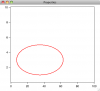# Generate a Rotatable EllipseI want to generate a ellipse of defined parameters on Cartesian coordiates and then be able to rotate it. Seems easy enough. To this point, I can generate an standard ellipse with center at (xo,yo) and major/minor axes of (a,b). My attempt to rotate it however changes the "width" and "height" of the ellipse while it rotates. I want a pure rotation operation.

Here is the basic Ellipse code I have developed, where yw and xw are waves of 361 points.

Function Ellipsoid(xo,yo,a,b,alpha)
variable xo,yo,a,b,alpha

//  wave yw  Assume these two waves exist .....
//  wave xw

variable sinbeta = sin(-alpha*Pi/180), cosbeta=cos(-alpha*Pi/180)

xw = xo + a*cos(p*Pi/180)*cosbeta - b*sin(p*Pi/180)*sinbeta
yw = yo + a*cos(p*Pi/180)*sinbeta + b*sin(p*Pi/180)*cosbeta

return (0)
end

A plot of yw versus xw shows the ellipse.

I should also mention, the ellipse is to be drawn on linear or log-log coordinates. One intent is to show how an elliptical region spanning multiple decades in scale in linear coordinates takes on a completely different shape when displayed in log-log coordinates. Anyone who is familiar with materials selection charts that are popular in books by M. Ashby and others will see the immediate pedagogical utility of my efforts.

I have attached an experiment to illustrate where I am so far.

Can anyone suggest a better way to do what I need?Thank you. This helped some. However, the matrix rotation operation still skews the ellipse just as my operation was doing (it was in fact the same thing). So, something is still fundamentally incorrect in my understanding and implementation.

I'll just leave rotation off for now.

--
J. J. Weimer
Chemistry / Chemical & Materials Engineering, UAH
jjweimer wrote:
However, the matrix rotation operation still skews the ellipse just as my operation was doing (it was in fact the same thing). So, something is still fundamentally incorrect in my understanding and implementation.

Rotation is a unitary operation, therefore keeping the distances between points constant - that's why your ellipse, if your code is correct, cannot skew. In fact, your code is OK, and your ellipse does NOT skew, this happens only because of different scales on the x- and y-axis. If you fix the scales to be the same, you will see that your function works as expected.

Cheers,
gregor
gregorK wrote:
Rotation is a unitary operation, therefore keeping the distances between points constant - that's why your ellipse, if your code is correct, cannot skew.

Oh! Duh! Of course. My mistake was certainly in forgetting that my axes were not isomorphic in scale. Rotation therefore works as expected. The code snippet is found here.

Thank you!

--
J. J. Weimer
Chemistry / Chemical & Materials Engineering, UAH
You can try my ellipse-drawing package by putting this line into your Procedure window:
#include <DrawEllipseOnGraph>

That will add a submenu to your Graph menu. It provides an interactive way to draw an ellipse with draggable handles to change the aspect ratio and angle.

John Weeks
WaveMetrics, Inc.
support@wavemetrics.com
johnweeks wrote:
You can try my ellipse-drawing package by putting this line into your Procedure window:

#include <DrawEllipseOnGraph>

That will add a submenu to your Graph menu. It provides an interactive way to draw an ellipse with draggable handles to change the aspect ratio and angle.

I will look into it. My direct interest is to draw an ellipse of a given aspect/angle a) in normal coordinates and then transform to log-log to see the distortion in shape and b) in log-log coordinates and then transform to normal coordinates to see the distortion in shape. This is all related to illustrating the basics of so-called "materials selection charts" where ellipses are used on log-log plots to map out inclusive regions for two coordinating properties (strength vs density for example) of certain classes of materials (metals, composites ....). Showing that a pure ellipse in one coordinate framework is not a pure ellipse in another is a fundamental part of understanding such charts.
--
J. J. Weimer
Chemistry / Chemical & Materials Engineering, UAH
jjweimer wrote:
My direct interest is to draw an ellipse of a given aspect/angle a) in normal coordinates and then transform to log-log to see the distortion in shape and b) in log-log coordinates and then transform to normal coordinates to see the distortion in shape.

Woops. My package doesn't handle log axes...

John Weeks
WaveMetrics, Inc.
support@wavemetrics.com